# Partial fields, II. The Lift Theorem

A long time ago, I wrote about partial fields. I defined a partial field as a pair $\mathbb{P} = (R, G)$ of a commutative ring $R$ and a subgroup $G$ of the units of $R$, such that $-1 \in G$. I defined a $\mathbb{P}$-matrix as a matrix over $R$ such that every square sub matrix has a determinant in the set $G \cup \{0\}$, and showed that there is an associated matroid $M[A]$ if some $r\times r$ sub matrix has nonzero determinant.

Partial fields first arose in Whittle’s study of matroids having representations over several different fields. In this post I will discuss the main results of his work on ternary matroids [Whi95, Whi97]. First, let me remind you of Tutte’s characterization of the regular matroids:

Theorem 1. The following statements are equivalent for a matroid $M$:

1. $M$ is representable over both $\text{GF}(2)$ and $\text{GF}(3)$;
2. $M$ is representable over $\text{GF}(2)$ and some field of characteristic other than $2$;
3. $M$ is representable over $\mathbb{R}$ by a totally unimodular matrix;
4. $M$ is representable over every field.

In addition, Tutte showed that there are exactly three excluded minors for the class of regular matroids: $\{U_{2,4}, F_7, F_7^*\}$. If you’ve never done so, you should definitely read Bert Gerards’ concise proof of this fact [Ger89]. Geoff Whittle wondered if similar theorems would hold when the set of fields in the fourth outcome were changed. In particular, what if we don’t require the matroid to be binary? Here is one of his theorems:

Theorem 2. The following statements are equivalent for a matroid $M$:

1. $M$ is representable over both $\text{GF}(3)$ and $\text{GF}(5)$;
2. $M$ is representable over all fields of characteristic other than $2$;
3. $M$ is representable over $\mathbb{Q}$ by a matrix with every square submatrix in $\{0\} \cup \{\pm 2^i : i \in \mathbb{Z}\}$.

Recall from the last post the dyadic partial field $\mathbb{D} = (\mathbb{Z}[\frac{1}{2}], \{\pm 2^i : i \in \mathbb{Z}\})$. Let $\mathbb{F}$ be a field of characteristic $p$ other than 2, and define the ring homomorphism $\phi: \mathbb{Z}[\frac{1}{2}] \to \mathbb{F}$ by sending $x \in \mathbb{Z}$ to $x \pmod p$ and $\frac{1}{2}$ to the inverse of $\phi(2)$ in $\mathbb{F}$. Then $\phi$ is a partial-field homomorphism, and therefore any dyadic matrix $A$ will have $M[A] = M[\phi(A)]$. With this homomorphism, the implication $(3) \implies (2)$ follows immediately. The implication $(2) \implies (1)$ is trivial. To show that $(1)$ implies $(3)$ we need some more work.

fundamental element of a partial field $\mathbb{P}$ is an element $p \in G$ such that $1-p \in G$ as well. Together with Rudi Pendavingh I proved the following theorem, generalizing Whittle’s proof techniques:

Theorem 3. Let $\mathbb{P}_1$ and $\mathbb{P}_2$ be partial fields, and $\phi: \mathbb{P}_1\to \mathbb{P}_2$ a partial-field homomorphism whose restriction to the fundamental elements of $\mathbb{P}_1$ and $\mathbb{P}_2$ is a bijection. Let $A_2$ be a $\mathbb{P}_2$-matrix. Then exactly one of the following holds:

1. There exists a $\mathbb{P}_1$-matrix $A_1$ such that $\phi(A_1) = A_2$;
2. $A_2$ can be transformed, using pivots, row and column permutations, transposing, and row and column scaling, to a matrix $A$ of the form $$\begin{bmatrix} 1 & 1 & 1\\ 1 & a & b\end{bmatrix} \textit{ or } \begin{bmatrix} 1 & 1 & 0 & 1\\ 1 & 0 & 1 & 1\\ 0 & 1 & 1 & 1 \end{bmatrix}$$ such that (1) does not hold for $A$ either.

The associated matroid is $M[I\ A_1]$. To use this theorem for Whittle’s result, let $\mathbb{P}_1 = \mathbb{D}$ and $\mathbb{P}_2 = \text{GF}(3)\times \text{GF}(5)$, where addition and multiplication are componentwise. Then all we have to check is that there is a bijection between the matrices of the forms above between these two partial fields, which is a finite task (because the entries $a$ and $b$ have to be fundamental elements). We called this result the lift theorem, because it allows us to “lift” the finite representation over the partial field $\text{GF}(3) \times \text{GF}(5)$ to a representation over the infinite partial field $\mathbb{D}$.

The proof of the theorem follows Gerards’ proof of Tutte’s theorem (mentioned above) very closely. A new ingredient, though, is the construction of the matrix $A_1$ out of the matrix $A_2$. I will briefly sketch this construction. Let $G= (V, E)$ be the bipartite graph whose vertices are the rows and columns of the matrix $A_2$, and whose edges correspond to the nonzero entries. We may scale so that the entries corresponding to a spanning tree $H$ of $G$ are 1. We fill $A_1$ with $1$s corresponding to the spanning tree, $0$s corresponding to the zero entries of $A_2$, and question marks for the other entries. We repeatedly select a question mark for which the shortest path in $H$ between its terminal vertices is minimal. Up to row and column permutations, this matrix has the following shape: $$\begin{bmatrix} * & 0 & \cdots & 0 & *\\ * & * & 0 & & 0\\ 0 & * & \ddots & \ddots & \vdots\\ \vdots & \ddots & \ddots & * & 0\\ 0 & \cdots & 0 & * & * \end{bmatrix}$$ (where the top right entry corresponds to the question mark in $A_1$). We scale the rows and columns of both this sub matrix of $A_2$ and of $A_1$ to get the following pair:

$$\begin{bmatrix} -1 & 0 & \cdots & 0 & p\\ 1& -1 & 0 & & 0\\ 0 & 1 & \ddots & \ddots & \vdots\\ \vdots & \ddots & \ddots & -1 & 0\\ 0 & \cdots & 0 & 1 & -1 \end{bmatrix}, \begin{bmatrix} -1 & 0 & \cdots & 0 & ?\\ 1& -1 & 0 & & 0\\ 0 & 1 & \ddots & \ddots & \vdots\\ \vdots & \ddots & \ddots & -1 & 0\\ 0 & \cdots & 0 & 1 & -1 \end{bmatrix}.$$

The determinant of the left matrix is $\pm (1-p)$, so $p$ is a fundamental element of $\mathbb{P}_2$. Since there is a unique $q$ such that $\phi(q) = p$, we can replace the question mark with $q$. Then we revert the scaling, and add the corresponding edge to $H$.

We can show that, if a $\mathbb{P}_1$-matrix $A_1$ exists with $\phi(A_1) = A_2$, then this construction finds it. Moreover, the construction commutes with pivoting because it is unique up to row and column scaling. The rest of the proof roughly goes as follows:

Take a minimal counterexample $A_2$. We may assume the bipartite graph is connected and not a path or cycle. Possibly after transposing we can find two columns $u$ and $v$ such that $G – u, G – v, G – \{u,v\}$ are all connected. Scale as above, where the spanning tree has $u$ and $v$ as leaves. Find a matrix $A_1 – u$ corresponding to $A_2 – u$ (by which we mean the matrix with column $u$ removed), and a matrix $A_1 – v$ corresponding to $A_2 – v$. These matrices will agree on the columns other than $u, v$. If the matrix $A_1$ obtained by overlaying these two is not a $\mathbb{P}_1$-matrix, some determinant is wrong. By pivoting, we can ensure this determinant is $2\times 2$ and involves columns $u$ and $v$. Let $a,b$ be the rows of this sub matrix. In $G – u,v$ find a path from $a$ to $b$. More pivots result in a path of length $2$ or $4$, and a sub matrix of the following form (the $*$ symbols correspond to the bad sub matrix):

$$\begin{bmatrix} 1 & * & *\\ 1 & * & *\end{bmatrix} \textit{ or } \begin{bmatrix} 1 & 1 & p & q\\ 1 & 0 & * & *\\ 0 & 1 & * & * \end{bmatrix}$$

Then a little more analysis cuts down on the possible values of the entries.

Whittle also proved the following characterizations:

Theorem 4. The following statements are equivalent for a matroid $M$:

1. $M$ is representable over both $\text{GF}(3)$ and $\text{GF}(4)$;
2. $M$ is representable over all fields having a root of $x^2 – x + 1$;
3. $M$ is representable over $\mathbb{C}$ by a matrix with every square submatrix in $\{0\} \cup \{\zeta^i : i = 1, \ldots, 6\}$, where $\zeta$ is a complex sixth root of unity.

Theorem 5. The following statements are equivalent for a matroid $M$:

1. $M$ is representable over both $\text{GF}(3)$, $\text{GF}(4)$, and $\text{GF}(5)$;
2. $M$ is representable over both $\text{GF}(3)$ and $\text{GF}(8)$;
3. $M$ is representable over all fields with at least 3 elements;
4. $M$ is representable over $\mathbb{Q}(\alpha)$, where $\alpha$ is an indeterminate, by a matrix with every square submatrix in $\{0\} \cup \{\pm \alpha^i(1-\alpha)^j : i, j \in \mathbb{Z}\}$.

Theorem 6. The following statements are equivalent for a matroid $M$:

1. $M$ is representable over both $\text{GF}(3)$ and $\text{GF}(7)$;
2. $M$ is representable over $\text{GF}(3)$, $\text{GF}(p^2)$ for all $p > 2$, and over $\text{GF}(p)$ when $p \equiv 1 \pmod 3$;
3. $M$ is representable over $\mathbb{C}$ by a matrix with every square submatrix in $\{0\} \cup \{\pm 2^i\zeta^j : i,j \in \mathbb{Z}\}$.

Most excitingly, Whittle also managed to prove that these are all theorems of this kind involving $\text{GF}(3)$:

Theorem 7. Let $M$ be a matroid representable over $\text{GF}(3)$ and some field of characteristic other than 3. Then $M$ is representable over at least one of $\{\text{GF}(2), \text{GF}(4), \text{GF}(5), \text{GF}(7)\}$.

An analogous result for $\text{GF}(4)$ and other fields, unfortunately, cannot be obtained: because of the presence of the free spikes, which are representable over all sufficiently large prime fields, there is an infinite number of different classes of matroids representable over $\text{GF}(4)$ and $\text{GF}(p)$, as $p$ ranges over the primes.

Rudi Pendavingh recently implemented the lift construction mentioned above in Sage (it is part of the latest developer beta version). I will write about that in some future post.

References

[Ger89] Bert Gerards. A short proof of Tutte’s characterization of totally unimodular matrices. Lin. Alg. Appl. 114/115:207-212, 1989.

[Whi95]  Geoff Whittle. A characterisation of the matroids representable over GF(3) and the rationals. J. Combin. Theory Ser. B, 65(2):222–261, 1995.

[Whi97]  Geoff Whittle. On matroids representable over GF(3) and other fields. Trans. Amer. Math. Soc., 349(2):579–603, 1997.

# A menagerie of infinite matroids

So far in this series we have focused on the search for a good way to axiomatise infinite matroids. Now that we have the axioms, our next aim is to get a better sense of what sort of ground they stake out by taking a whirlwind tour of the known examples of infinite matroids.

Let’s start off with some very familiar examples: The set of subsets of a given set which have size at most $n$,  the set of (edge sets of) forests in a given graph and the set of linearly independent subsets of a given family of vectors in a vector space each give the set of independent sets of a matroid. This is true even if we allow the set, graph or family of vectors in question to be infinite. Of course, all of these examples are finitary (that is, a set is independent as soon as all of its finite subsets are). We saw earlier that it would be a bad idea to only consider finitary matroids, because we would lose the power of duality. But we certainly want our notion of infinite matroid to include all of these examples. Since we now have a concept of infinite matroid which is closed under duality, we get all their duals for free, too.

What about matroids which are neither finitary nor cofinitary? At first it looks like a simple way to construct such examples would be by building infinite uniform matroids. But the usual definition of uniformity, namely that the bases should be all sets of a given size, becomes silly when we replace size’ by cardinality’, since infinite sets can have proper subsets of the same cardinality. It doesn’t help to define things in terms of independent sets. For example, we might hope to have a matroid whose independent sets are just the countable subsets of some uncountable set $E$. But since there are no maximal countable sets, this object wouldn’t even have any bases.

The right notion of uniformity for infinite matroids is as follows: if $B$ is a base and $x$ and $y$ are elements of the ground set $E$ with $x$ in $B$ but $y$ not in $B$ then $B – x + y$ is also a base. It isn’t hard to check that this is equivalent to uniformity when applied to finite matroids. Now we might try to build infinite uniform matroids by just recursively choosing the set of bases, paying attention to the fact that (because of the definition above) whenever we choose a set $B$ we also have to choose all sets $B’$ for which $B \setminus B’$ and $B’ \setminus B$ are finite and of equal size. This simpleminded strategy works, given some weak assumptions [BG15]. Because this recursive construction gives us so much freedom, infinite uniform matroids are much more varied than finite ones.

The flexibility of this construction lets us resolve a very basic question: must it be true that any two bases of an infinite matroid have the same cardinality? Higgs showed that this is true if we assume the continuum hypothesis [H69a]. But using the freedom mentioned above we can also show that it is consistent that the following naive plan for building a matroid with bases of different sizes actually works: run the above recursive construction to build a uniform matroid on an uncountable set, and begin by nominating some countable subset and some uncountable subset as bases [BG15]. So the answer to the question above is actually independent of the usual axioms of set theory.

The other examples we’ll look at today will all be associated to graphs. The finitary matroid mentioned above, whose circuits are (edge sets of) cycles in a given graph $G$, is called the finite-cycle matroid of $G$. There is another finitary matroid whose circuits are the finite bonds of $G$. But these two are no longer dual to each other. The circuits of the dual of the finite-bond matroid correspond to topological circles in a topological space, called the end-compactification of $G$. This space is obtained from $G$ by adding some points at infinity, called ends. These `topological cycles’ were actually first invented for a very different reason and helped to motivate the current axiomatisations of infinite matroids. This was the starting point of our story.

So although there is one canonical matroid associated to a finite graph, for an infinite graph we already have two. In fact there are many more. For example, Higgs showed that there very often is a matroid whose circuits are the (edge sets of) finite cycles or double rays in the graph $G$. This doesn’t always work. For example, in the Bean Graph, shown below, the set of bold edges and the set of dashed edges are both bases but there is no way to replace the edge $e$ with a bold edge in the dashed basis and still have a base. So the base axioms don’t hold.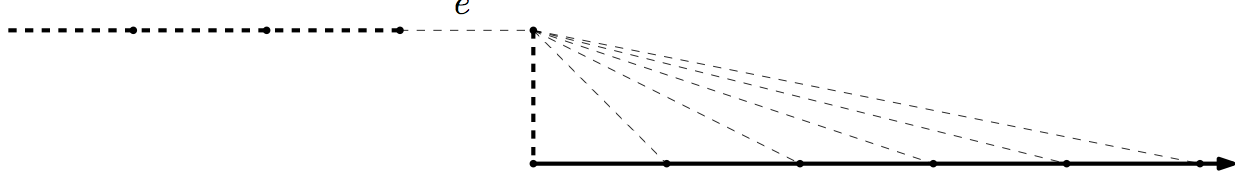This is essentially all that can go wrong: Higgs showed [H69b] that if $G$ includes no subdivision of the Bean Graph then there is a matroid, called the algebraic-cycle matroid of $G$, whose circuits are given as above by the finite cycles and double rays.

Another fertile way to build more matroids from graphs is to ignore some of the ends. More precisely, we consider topological circles in a space obtained from the end-compactification of $G$ by deleting some of the ends. For example, the double ladder has two ends and we might wish to keep only one of them, giving rise to the following space: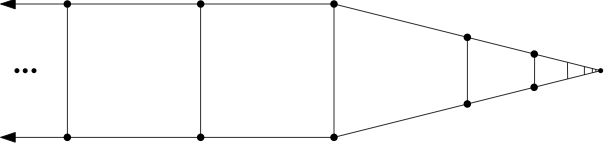Once more it is true that the (edge sets of) topological circles give the circuits of a matroid, as long as the set of ends we remove is Borel [BC15].

Is there any unifying theme to this profusion of examples, except that they can all be built somehow from graphs? Yes! Each of these matroids can represented by a topological space. The finite-cycle matroid of $G$ is represented by the geometric realisation of $G$ and the topological-cycle matroid by the end-compactification. The rich collection of matroids we just discussed are represented by subspaces of the end-compactification. The algebraic-cycle matroid is represented by the space obtained from the end-compactification by identifying all the ends.

More generally, there is a precisely specified class of graph-like topological spaces and a  notion of representation of a matroid by such a space, such that a matroid is representable in this way if and only if, firstly, every intersection of a circuit with a cocircuit is finite (this corresponds to the fact that topological circles are compact), and secondly, it satisfies the usual excluded-minor characterisation of graphic matroids.

Thus the wide range of ways of building infinite matroids from graphs corresponds to the variety of ways of building such graph-like spaces out of graphs. We have seen that this construction doesn’t always give a matroid, as with the Bean Graph above, but it does so often enough to give a rich variety of matroids. For example, we always get a matroid when the space is compact. Hence the (edge sets of) topological circles in the following picture give the circuits of a matroid, the Sierpinski Matroid: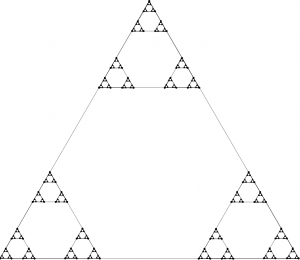Before we conclude, lets just glance at a couple of the other kinds of infinite matroid which have been discovered. There are frame matroids: Matthews and Oxley showed that in any graph $G$ the edge sets of subdivisions of the following graphs gives the set of circuits of a matroid [MO77], and a more general construction works in in infinite biased graphs.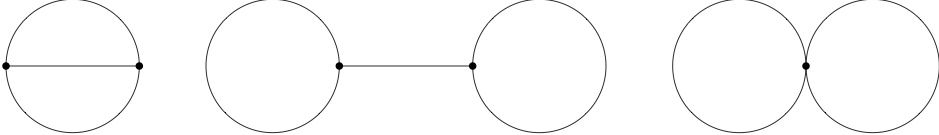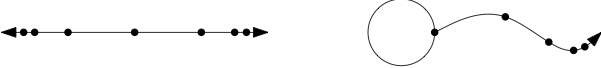There are infinite gammoids, defined either in terms of bipartite graphs or of directed graphs. The question of when these constructions give rise to matroids is a delicate one and has been investigated by Afzali, Law and Müller [ALM15].

Finally, we can define the truncation of a non-free matroid $M$ to have as independent sets the proper subsets of independent sets of $M$, and all truncations and co-truncations of infinite matroids are again infinite matroids. This gives lots of new examples, such as the matroids whose circuits are edge sets of topological copies of the following spaces in a graph-like space: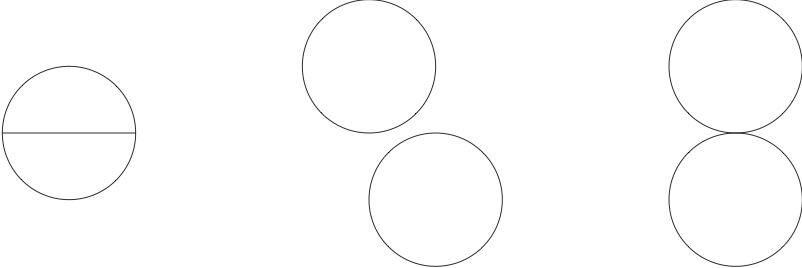This is not so much a class of examples as a way to build new infinite matroids from old. We’ll consider some other fertile ways to combine infinite matroids next time.

[ALM15] H. Afzali, Hiu-Fai Law and M. Müller, Infinite gammoids. Electronic J. Comb. 22 (2015), #P1.53
[BC15] N. Bowler and J. Carmesin, Infinite Matroids and Determinacy of Games, submitted to LMS, available here.
[BG15] N. Bowler and S. Geschke,  Self-Dual Uniform Matroids on Infinite Sets, to appear in the Bulletin of the American Mathematical Society, available here.
[H69a] D. Higgs, Equicardinality of bases in B-matroids, Canadian Math. Bul. 12 (1969), 861–862.
[H69b] D. Higgs. Infinite graphs and matroids. Recent Progress in Combinatorics, Proceedings Third Waterloo Conference on Combinatorics, Academic Press (1969),  245-53.
[MO77] L. Matthews, J. Oxley, Infinite graphs and bicircular matroids. Discrete Mathematics, Volume 19, Issue 1 (1977), 61-65.